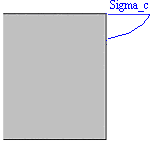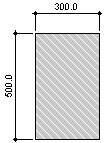# RF-CONCRETE Members – Online Manual Version 5

Online manuals, introductory examples, tutorials, and other documentation.

# 2.2.6 Creep and Shrinkage

### Creep and Shrinkage

Determination of initial values

This chapter gives an overview of the time-dependent stresses and strains due to creep and shrinkage. The influence of creep and shrinkage is used in the analytical serviceability limit state design for the determination of the deformation. The approach of creep and shrinkage in the nonlinear calculation is described in Chapter 2.4.6.

Creep is the time-dependent deformation of concrete under loading over a specific period of time. The essential influence values are similar to those of shrinkage, with the so-called creep-producing stress having considerable effects on the creep deformation. Special attention must be paid to the load duration, the point of time of load application, as well as to the extent of actions. The creep determining value is the creep coefficient φ (t, t0) at the relevant point of time t.

Shrinkage describes a time-dependent modification of volume without influence due to external loads or temperature. We will not elaborate on further expansion of the shrinkage problem into individual types (drying shrinkage, autogenous shrinkage, plastic shrinkage, and carbonation shrinkage). Significant influence values of shrinkage are relative humidity, effective thickness of structural components, aggregate, concrete strength, water-cement ratio, temperature, as well as the type and duration of curing. The shrinkage determining value is the shrinkage strain εc,s (t, ts) at the relevant point of time t.

Hereafter, the determination of the creep coefficient φ (t, t0) and shrinkage strain εc,s (t, ts) according to EN 1992-1-1, clause 3.1.4 and annex B is described.

Creep coefficient φ (t, t0)

Using the following formulas requires the creep-producing stress σc of the acting permanent load to not exceed the following value:

where

• fckj : cylinder compressive strength of concrete at point of time when creep-producing stress is appliedFigure 2.5 Creep-producing stress

Under the assumption of a linear creep behavior (σc ≤ 0.45 ⋅ fckj), the concrete's creep can be determined by a reduction of the modulus of elasticity for concrete.

where

• Ecm : mean modulus of elasticity according to EN 1992-1-1, Table 3.1
• φeff (t, t0) : effective creep coefficient, φeff (t, t0) = φ (t, t0) ⋅ MQP / MEd
• t : age of concrete in days at relevant point of time
• t0 : age of concrete in days when load application starts

The creep coefficient φ (t, t0) at the analyzed point of time t may be calculated as follows:

where

• RH : relative humidity in [%]
• h0 : effective thickness of structural component in [mm]
• h0 = 2 ∙ Ac / u
• Ac : cross-sectional area
• u : cross-section perimeter
• α1, α2 : adjustment factors
• α1 = (35 / fcm)0.7
• α2 = (35 / fcm)0.2
• fcm : mean value of cylinder compressive strength

• fcm : mean value of cylinder compressive strength of concrete in [N/mm2]

• t0 : age of concrete in days when load application starts

• t : age of concrete in days at relevant point of time
• t0 : age of concrete in days when load application starts
• βH = 1.5 ⋅ [1 + (0.012 ⋅ RH)18] ⋅ h0 + 250 ⋅ α3 ≤ 1500 ⋅ α3
• RH : relative humidity in [%]
• h0 : effective thickness of structural component [mm]
• α3 : adjustment factor
• α3 = (35 / fcm)0.5 ≤ 1.0

The following input is required to calculate the creep coefficient:

• RH : relative humidity in [%]
• t0 : age of concrete in days when load application starts
• t : age of concrete in days at relevant point of time (optionally ∞)

The influence of high or low temperature ranging from 0°C to 80°C on the concrete's maturity can be taken into account by correcting the concrete age with the following equation:

where

 n number of periods with identical temperature T (Δti) temperature in [°C] during time period Δti Δti number of days with this temperature T

The influence of the type of cement on the concrete's creep coefficient can be taken into account by modifying the load application age t0 with the following equation:

where

 t0,T = tT effective age of concrete when load application starts considering influence of temperature α exponent, depends on type of cement, see Table 2.2
Table 2.2 Exponent α
α Type of Cement

−1

slow-hardening cements of class S

0

normal- or rapid-hardening cements of class N

1

rapid-hardening, high-strength cements of class R

ExampleFigure 2.6 concrete C25/30cement CEM 42.5 NRH: 50%Two temperature changes:6 days - temperature 15 °C8 days - temperature 7 °CConsidered age of concrete tk: 365 days

Age of concrete when creeping starts:

Age of concrete under influence of type of cement:

Effective structural component thicknesses:

Creep coefficient:

where

Coefficient of shrinkage ε (t, ts)

When determining the coefficient of shrinkage ε (t, ts) according to EN 1992-1-1, clause 3.1.4, the shrinkage strain εcs (t) can be calculated from the sum of the components of the autogenous shrinkage εca (t) and drying shrinkage εcd (t, ts).

The autogenous shrinkage strain εca at the relevant point of time (t) is determined as follows:

where

The component from drying shrinkage εcd is determined as follows:

where

• t age of concrete in days at relevant point of time
• ts age of concrete in days when shrinkage starts
• h0 effective cross-section thickness in [mm] : h0 = 2 ⋅ Ac / u

• fcm : mean cylinder compressive strength of concrete in [N/mm2]
• fcm0 : 10 N/mm2
 Cement class Property αds1 αds2 S slow-hardening 3 0.13 N normal-hardening 4 0.12 R rapid-hardening 6 0.11

• RH relative humidity of environment in [%]
• RH0 100 %
Example

concrete C25/30

cement CEM 42.5 N

RH: 50 %

Age of concrete ts when shrinkage starts: 28 days

Considered age of concrete t: 365 days

Effective cross-section thickness:

Autogenous shrinkage:

where

Drying shrinkage:

where

Cement class N ⇒ αds1 = 4; αds2 = 0.12

Total coefficient of shrinkage: